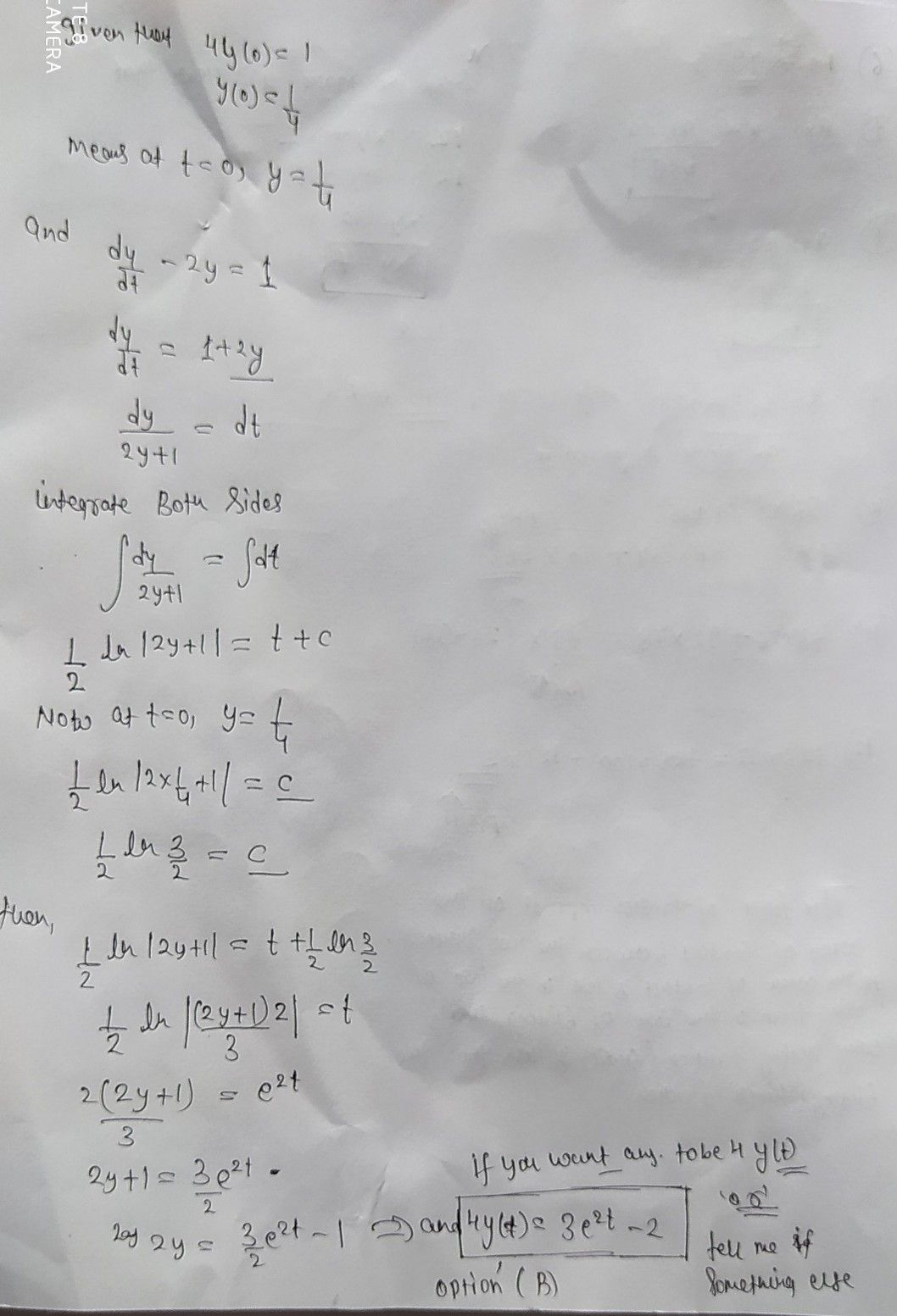Symbol
Problem$1fy\left(t\right)$ is the solutionm of $\dfrac {4y} {a}-2y=4y\left(0\right)=1,$ then $2$ $y\left(t\right)$ $=$ $\left(t\right)2a^{x}-2$ $x-2$ $33$ $22y$ $-3$ $10\right)$ $\left(0\right)2c^{2}-2$
Other
Search count: 117
SolutionQanda teacher - AskKavyaIf you have any doubt feel free to ask:) Dear, Please evaluate the answer with 5 star and give gift coins(it does not reduce your coin balance.) for giving gift coins Click on evaluate. Then press the + icon 3 times(3000).